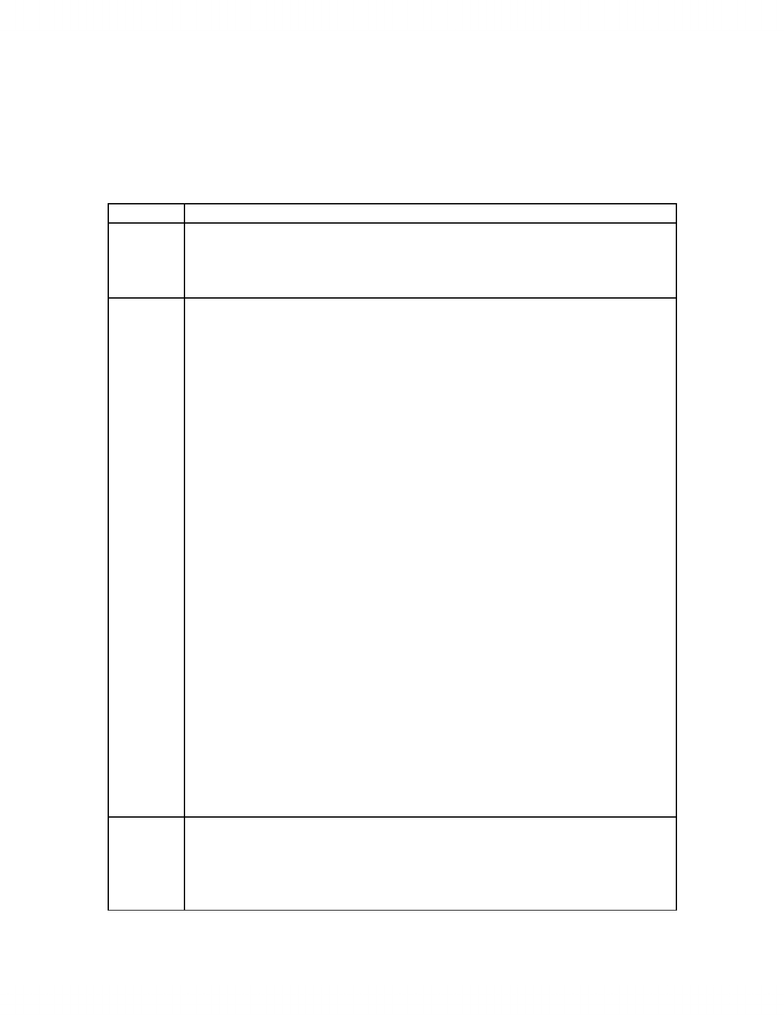# Notes taken during lecture

41 views4 pages
School
Department
Course
ProfessorEXAM: 3 hours; 5 short answers, 28 M/C questions
-half of exam is new material (untested)
-other half is units 1 to 4
LECTURE 14
Slide No. Notes
-electrochemistry deals with relationship between chemical
reactions and electricity
-spontaneous reactions can produce electricity and electricity can
cause non-spontaneous reactions to occur
1-Delta S is negative because entropy of gas greater than that of
liquids
-Spontaneity – calculate delta G
-H and O gas both 0
-Therefore spontaneous
-Delta G = Delta H etc. formula
-Both delta H and delta S are negative
-Whenever both signs the same, some temperatures spontaneous
some not
-Reaction becomes non-spontaneous at high temperatures
-To calculate K for the reaction…
-Delta G = -RTInK
-Q = 1/ (P of H2)squared times P of O2 = 1
-Therefore K is greater than one for reaction to go to products
-Therefore answer either E or D
-To actually determine K
-(1000J/kJ)(-474kJ) = (-8.314J/molK)(298K)InK
-K = 1.44x10^83 therefore reaction goes to completion
-Ans: E
-To calculate temperature when reaction non-spontaneous
-Calculate temperature when reaction switches from non-
spontaneous to spontaneous
-Delta G = Delta H – T times delta S with delta G = 0
-Delta H = 2mol(-285.8kJ/mol) = -571.6kJ
-Delta S = 2mol(699.9J/molK) – 2mol(130.6J/molK)
1mol(205J/molK) = -326.4J/K
-T = delta H/delta S = 1751 K
-Reaction is non-spontaneous at temperatures above 1751K
2-LEO: losing electrons is oxidation; GER: gaining electrons is
reduction
-OIL RIG
-overall reaction – electrons cancel out
-
www.notesolution.com
Unlock document

This preview shows page 1 of the document.
Unlock all 4 pages and 3 million more documents.

## Document Summary

Exam: 3 hours; 5 short answers, 28 m/c questions half of exam is new material (untested) other half is units 1 to 4. Notes electrochemistry deals with relationship between chemical reactions and electricity spontaneous reactions can produce electricity and electricity can cause non-spontaneous reactions to occur. Delta s is negative because entropy of gas greater than that of liquids. Delta g = delta h etc. formula. Both delta h and delta s are negative. Whenever both signs the same, some temperatures spontaneous some not. Q = 1/ (p of h2)squared times p of o2 = 1. Therefore k is greater than one for reaction to go to products. K = 1. 44x10^83 therefore reaction goes to completion. Calculate temperature when reaction switches from non- (1000j/kj)(-474kj) = (-8. 314j/molk)(298k)ink spontaneous to spontaneous. Delta g = delta h t times delta s with delta g = 0. Delta s = 2mol(699. 9j/molk) 2mol(130. 6j/molk) . T = delta h/delta s = 1751 k.

## Get access

\$8 USD/m\$10 USD/m
Billed \$96 USD annuallyHomework Help
Study Guides
Textbook Solutions
Class Notes
Textbook Notes
Booster Class